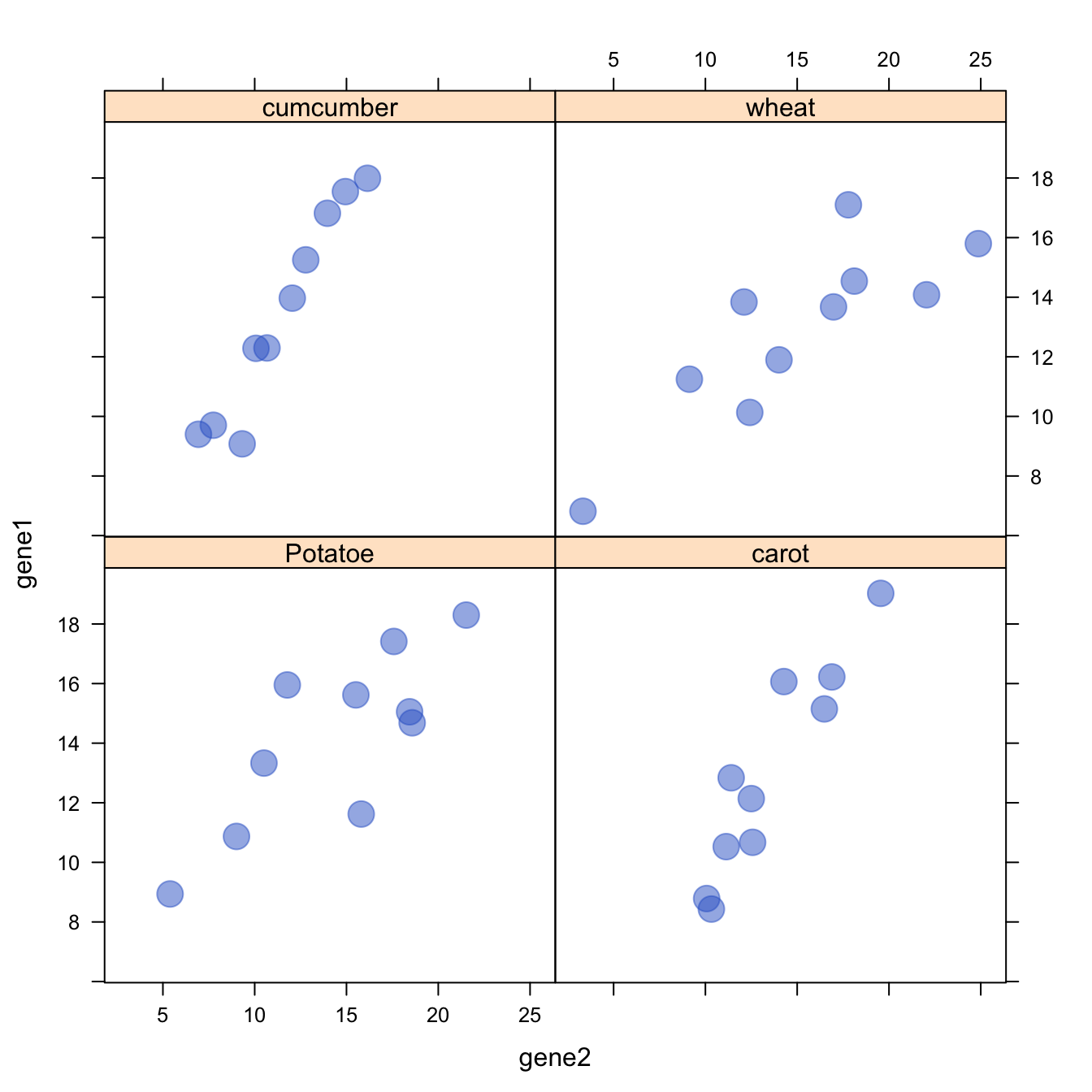# Lattice XY plot function

The `xyplot()` function of the `lattice` package allows to build a scatterplot for several categories automatically.

The `lattice` library offers the `xyplot()` function. It builds a scatterplot for each levels of a factor automatically.

It is actually the ancestor of the `geom_wrap()` function of ggplot2 than you can see in action here.``````# Library
library(lattice)

# create data :
sample <- paste(rep("sample_",40) , seq(1,40) , sep="")
specie <- c(rep("carot" , 10) , rep("cumcumber" , 10) , rep("wheat" , 10) , rep("Potatoe" , 10) )
gene1 <- c( seq(5,14)+rnorm(10 , 4 , 1) , seq(5,14)+rnorm(10 , 4 , 1) , seq(5,14)+rnorm(10 , 4 , 1) , seq(5,14)+rnorm(10 , 4 , 1) )
gene2 <- c( seq(5,14)+rnorm(10 , 4 , 1) , seq(5,14)+rnorm(10 , 2 , 0.2) , seq(5,14)+rnorm(10 , 4 , 4) , seq(5,14)+rnorm(10 , 4 , 3) )
data <- data.frame(sample,specie,gene1,gene2)

# Make the graph
xyplot(gene1 ~ gene2 | specie , data=data , pch=20 , cex=3 , col=rgb(0.2,0.4,0.8,0.5) )``````

Related chart types

## Contact

This document is a work by Yan Holtz. Any feedback is highly encouraged. You can fill an issue on Github, drop me a message on Twitter, or send an email pasting yan.holtz.data with gmail.com.